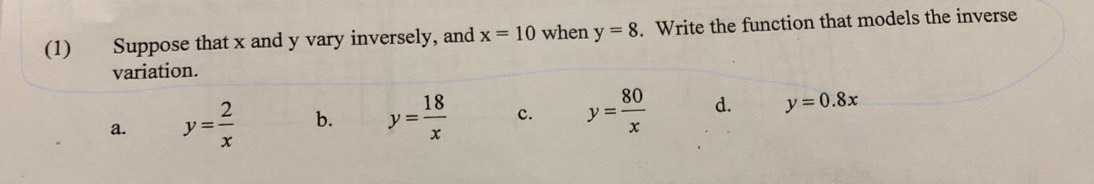### ¿Todavía tienes preguntas de matemáticas?

Pregunte a nuestros tutores expertos
Algebra
Pregunta(1) Suppose that $$x$$ and $$y$$ vary inversely, and $$x = 10$$ when $$y = 8$$ . Write the function that models the inverse variation. a. $$y = \frac { 2 } { x }$$ b. $$y = \frac { 18 } { x }$$ c. $$y = \frac { 80 } { x }$$ d. $$y = 0.8 x$$

c)$$y= \frac{80}{x}$$